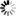Normal view

# Numerical methods : using MATLAB / G.R. Lindfield, J.E.T. Penny

Material type:TextPublication details: c2012 Edition: 3. edDescription: xviii, 534 p. ill. 24 cmISBN: 9780123869425 (pbk.)DDC classification: 518.028553 Other classification: 518 | Tak
Contents:
Machine generated contents note: An Introduction to Matlab Linear Equations and Eigensystems Solution of Non-linear Equations Differentiation and Integration Solution of Differential Equations Boundary Value Problems Fitting Functions to Data Optimization Methods Applications of the Symbolic Toolbox Appendix A - Matrix Algebra Appendix B - Error Analysis
Star ratingsAverage rating: 0.0 (0 votes)
Holdings
Item type Current library Call number Status Date due Barcode
Book (loan)
518 Available 080041485928
Book (loan)
518 Available 080041485929

Penny's name appears first on the earlier edition

Includes bibliographical references (p. 521-523) and index

Machine generated contents note: An Introduction to Matlab Linear Equations and Eigensystems Solution of Non-linear Equations Differentiation and Integration Solution of Differential Equations Boundary Value Problems Fitting Functions to Data Optimization Methods Applications of the Symbolic Toolbox Appendix A - Matrix Algebra Appendix B - Error Analysis

Adress: Biblioteket, Blekinge Tekniska Högskola, 371 79 Karlskrona
Telefon: 0455 - 38 51 01
E-post: biblioteket@bth.se
Ansvarig för sidan: Biblioteket